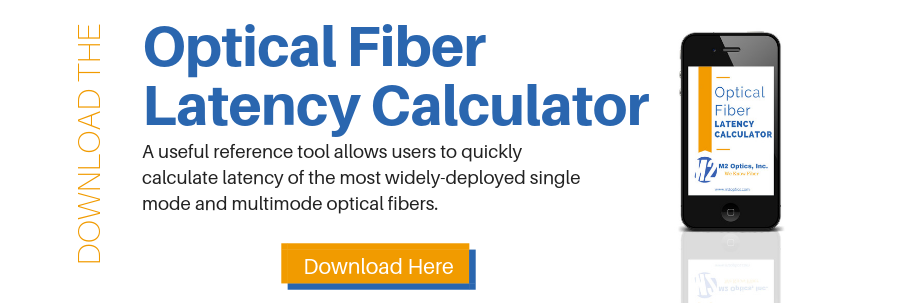<img alt="" src="http://www.oita4bali.com/151621.png" style="display:none;">

Latency is a term that is used to describe a time delay in a transmission medium such as a vacuum, air, or a fiber optic waveguide.  In free space, light travels at 299,792,458 meters per second.  This equates to 299.792 meters per microsecond (µs) or 3.34µs per kilometer.  In fiber optics, the latency of the fiber is the time it takes for light to travel a specified distance through the glass core of the fiber.  Light moving through the fiber optic core will travel slower than light through a vacuum because of the differences of the refractive index of light in free space and in the glass.

In a fiber optic waveguide, it is necessary to know the refractive index of the glass in the core before the speed of light (and thus latency) in the core can be calculated.  Since the refractive index of light in the fiber core is dependent on the slight variations of the refractive index of the glass at different wavelengths, it is necessary to know the wavelength being used in the system as well as the refractive index at that wavelength.

To demonstrate this, we used two types of single mode optical fibers (G.652 and G.655) from two leading manufacturers that are commonly deployed in networks around the world.   The wavelength dependent refractive index values shown in the table below are based on their published specifications:

 Optical Fiber Type Wavelength Refractive Index Distance* Brand A (G.652) 1310 nm 1.4677 204.260 m/µs 1550 nm 1.4682 204.191 m/µs Brand A (G.655) 1550 nm 1.468 204.218 m/µs 1625 nm 1.469 204.079 m/µs Brand B (G.652) 1310 nm 1.467 204.357 m/µs 1550 nm 1.468 204.220 m/µs Brand B (G.655) 1550 nm 1.470 203.940 m/µs 1625 nm 1.470 203.940 m/µs

* Distance = Speed of Light (299.792m/µs) / Refractive IndexFor example, using a 100km length of Brand B (G.652) fiber with a 1310nm laser would result in a latency of 489.34µs (100,000/204.357 = 489.34).  At 1550nm, the same fiber length would have a latency of 489.67µs (100,000/204.220 = 489.67).  A small difference of only 0.33 microseconds occurs as the result of using the two different wavelengths in the same fiber.

Note that only in the longest, most sensitive applications are the minor variations in refractive index important.  A refractive index of 1.47 can typically be used to calculate the latency or time delay of a fiber length to a generally acceptable accuracy.

Using all this information, a rule of thumb for quickly calculating latency in single mode fiber is using 4.9 microseconds per kilometer with 1.47 as the refractive index.  With a little less accuracy, you can use 5 microseconds per kilometer as a rounded guide for ease of calculation in determining overall latency of a known length fiber link.